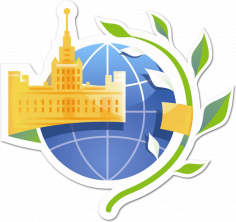Date
8 - 12 Apr 2019

# Lomonosov 2019

International scientific conference of students and young scientists "Lomonosov"

City
Moscow, Russia
Venue
MSU
Registration
Registration is closed

# International scientific conference of students and young scientists "Lomonosov"

Section "Mathematics and mechanics"

Section "Mathematics and mechanics"

Subsections

• Real, complex and functional analysis
• Differential equations, dynamical systems and optimal control
• Geometry and topology
• Probability theory and mathematical statistics
• Mathematical logic, algebra and number theory
• Computational mathematics, mathematical modeling and numerical methods
• Discrete mathematics and mathematical cybernetics
• Theory and methods of teaching mathematics
• Aeromechanics
• Computational mechanics
• Gas and wave dynamics
• Hydromechanics
• Mechanics of composites
• Applied mechanics and control
• Theoretical mechanics and mechatronics
• Theory of plasticity
• Theory of elasticity